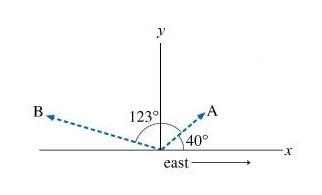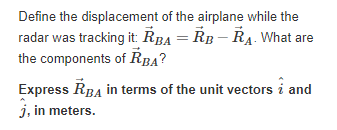# Question Solved1 AnswerA radar station, located at the origin of xy plane, as shown in (Figure 1), detects an airplane coming straight at the station from the east. At first observation (point A), the position of the airplane relative to the origin is R⃗ A. The position vector R⃗ A has a magnitude of 360 mm and is located at exactly 40 degrees above the horizon. The airplane is tracked for another 123 degrees in the vertical east-west plane for 5.0 s, until it has passed directly over the station and reached point B. The position of point B relative to the origin is R⃗ B (the magnitude of R⃗ B is 880 mm). The contact points are shown in the diagram, where the x axis represents the ground and the positive y direction is upward. y B 123 40° .X east Define the displacement of the airplane while the radar was tracking it: RBA = RB - RA. What are the components of ŘBA? Express ŘBA in terms of the unit vectors i and j, in meters.A radar station, located at the origin of xy plane, as shown in (Figure 1), detects an airplane coming straight at the station from the east. At first observation (point A), the position of the airplane relative to the origin is R⃗ A. The position vector R⃗ A has a magnitude of 360 mm and is located at exactly 40 degrees above the horizon. The airplane is tracked for another 123 degrees in the vertical east-west plane for 5.0 s, until it has passed directly over the station and reached point B. The position of point B relative to the origin is R⃗ B (the magnitude of R⃗ B is 880 mm). The contact points are shown in the diagram, where the x axis represents the ground and the positive y direction is upward.Transcribed Image Text: y B 123 40° .X east Define the displacement of the airplane while the radar was tracking it: RBA = RB - RA. What are the components of ŘBA? Express ŘBA in terms of the unit vectors i and j, in meters.
More
Transcribed Image Text: y B 123 40° .X east Define the displacement of the airplane while the radar was tracking it: RBA = RB - RA. What are the components of ŘBA? Express ŘBA in terms of the unit vectors i and j, in meters.